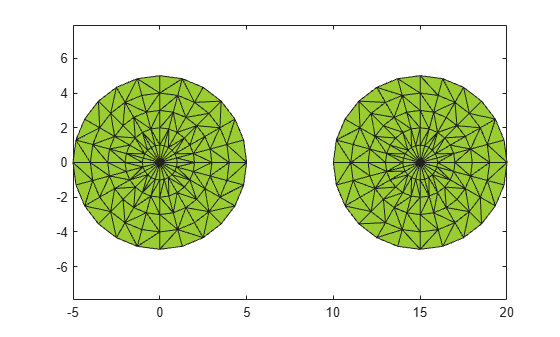nearestNeighbor

Determine nearest alpha shape boundary point

Description

I = nearestNeighbor(shp,qx,qy), for a 2-D alpha shape shp, returns the indices of points on the boundary of shp closest to the query points. I is the array of nearest neighbor indices where each index corresponds to the row index in shp.Points. The qx and qy query coordinates must be the same size.

I = nearestNeighbor(shp,qx,qy,qz), for a 3-D alpha shape, returns the indices of the boundary points of shp closest to (qx,qy,qz) and corresponds to the row indices in shp.Points. The qx, qy, and qz query coordinates must be the same size.

example

I = nearestNeighbor(shp,QP) specifies the query points as a matrix QP. For a 2-D alpha shape, QP is a matrix with two columns representing the qx and qy coordinates. For a 3-D alpha shape, QP has three columns representing the qx, qy, and qz coordinates.

I = nearestNeighbor(___,RegionID) returns the index of the nearest point that lies on the boundary of the region specified by RegionID, where 1RegionIDnumRegions(shp). You can include any of the input arguments in the previous syntaxes.

[I,D] = nearestNeighbor(___) additionally returns the Euclidean distance between the query point and its nearest neighbor. D has the same size as I.

Examples

collapse all

Create a set of 2-D points.

th = (pi/12:pi/12:2*pi)';
x1 = [reshape(cos(th)*(1:5), numel(cos(th)*(1:5)),1); 0];
y1 = [reshape(sin(th)*(1:5), numel(sin(th)*(1:5)),1); 0];
x = [x1; x1+15];
y = [y1; y1];

Create and plot an alpha shape with alpha radius equal to 1.

shp = alphaShape(x,y,1);
plot(shp)
hold onCompute the nearest shp boundary point to the query point QP. Plot the query point in blue and the nearest boundary neighbor in red.

QP = [6 3];
plot(QP(1),QP(2),'b.','MarkerSize',10)
hold on
I = nearestNeighbor(shp, QP);
plot(shp.Points(I,1),shp.Points(I,2),'r.','MarkerSize',10)Input Arguments

collapse all

Alpha shape, specified as an alphaShape object. For more information, see alphaShape.

Example: shp = alphaShape(x,y) creates a 2-D alphaShape object from the (x,y) point coordinates.

Query point x-coordinates, specified as a numeric array.

Data Types: double

Query point y-coordinates, specified as a numeric array.

Data Types: double

Query point z-coordinates, specified as a numeric array.

Data Types: double

Query point coordinates, specified as a two-column matrix or a three-column matrix.

• For 2-D, the columns of P represent qx and qy coordinates, respectively.

• For 3-D, the columns of P represent qx, qy, and qz coordinates, respectively.

Data Types: double

ID number for region in alpha shape, specified as a positive integer scalar between 1 and numRegions(shp).

An alpha shape can contain several smaller regions, depending on the point set and parameters. Each of these smaller regions is assigned a unique RegionID, which numbers the regions from the largest area or volume to the smallest. For example, consider a 3-D alpha shape with two regions. The region with the largest volume has a RegionID of 1, and the smaller region has a RegionID of 2.

Example: shp.RegionThreshold = area(shp,numRegions(shp)-2); suppresses the two smallest regions in 2-D alpha shape shp.

Data Types: double

Output Arguments

collapse all

Nearest neighbor indices, returned as an integer-valued array. The indices correspond to the row index of shp.Points and indicate the points on the boundary of shp that are closest to the given query points.

Distance from query points to nearest neighbors, returned as a numeric array. D is the 2-D or 3-D Euclidean distance and is the same size as I.